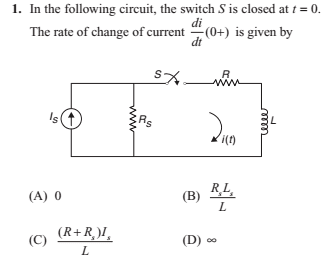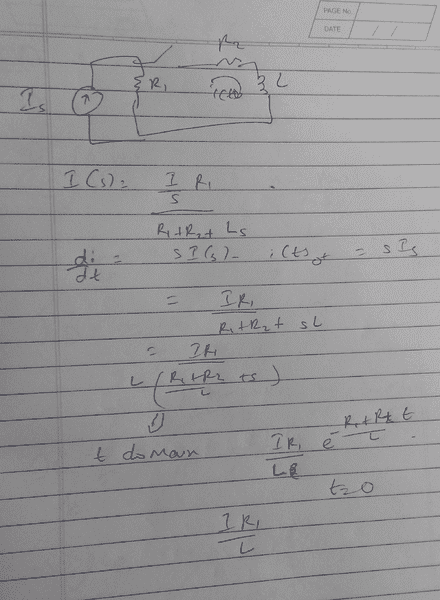# Rate of change of current in an R-L Circuit

• Engineering
• jaus tail
In summary, the conversation discusses the concept of current changing through an inductor and under what conditions this change can occur. The group discusses the use of source transformation and Laplace to arrive at the correct answer, and also clarifies the role of impulse voltage sources in this scenario. Ultimately, the conversation concludes that the current across an inductor cannot change suddenly unless the input is an impulse voltage source.

## Homework Statement## Homework Equations

Current cannot change suddenly through inductor unless input is impulse voltage source.

## The Attempt at a Solution

since input is DC source we have i(0+) = i(0-) so we have di/dt at t = 0+ is 0.
Is it correct? Book has mentioned answer as B which is weird cause there is no Ls in circuit.

#### Attachments

jaus tail said:
Book has mentioned answer as B which is weird cause there is no Ls in circuit.
It's probably a typo. They meant Is instead of Ls.

jaus tail said:
since input is DC source we have i(0+) = i(0-) so we have di/dt at t = 0+ is 0.
No.
How about using source transformation? It will make the circuit look more familiar.

•jaus tail
Wow... I used laplace and then got the answer. You're right.But shouldn't it be zero as per concept? Unless the input is an impulse voltage source the current across inductor cannot change so quickly. V = L di/dt. So unless V is an impulse, di/dt has to be zero.

#### Attachments

jaus tail said:
Wow... I used laplace and then got the answer. You're right.
Well, if you use source transformation, you end up with a series RL circuit with Rtotal=Rs+R and inductance L, excited by a voltage source of emf IsRs.
At t=0+, no current flows through the inductor, which means the entire source voltage appears across the inductor.
So, IsRs=Ldi/dt, which gives di/dt=IsRs/L.
jaus tail said:
the current across inductor cannot change so quickly. V = L di/dt. So unless V is an impulse, di/dt has to be zero.
No, here VL at t=0 is not zero, hence di/dt is also not zero. You can't compare the currents at t=0- and t=0+ to find di/dt at t=0+ because at t=0-, the inductor was not excited.

Well you're method is way quicker than mine.
Thanks a lot. Learnt new thing today.